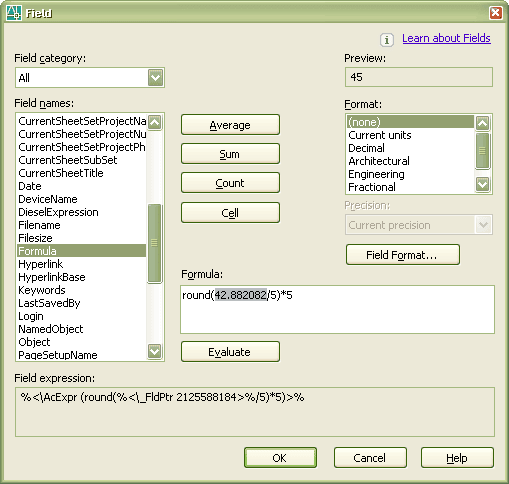## AutoCAD 2006 and formulas in fields, tables and the calculator

A great addition is formulas that can be used in fields, tables and the calculator. The question is what functions can be used? I've searched the documentation and found that the QuickCalc Calculator, CAL supports some standard numeric functions. Here is the list: abs,acos,asin,atan,cos,d2r,exp,exp10,ln,log,pi,r2d,round,sin,sqr,sqrt,tang,trunc as well as these operators: ^,*,/,+,-,(,). Even though it's not specifically documented these functions and operators can be used in formulas in fields and tables. When checking further on the formulas in fields I found that when you are editing a formula you can insert new fields. In the sample showed on the image I've grabbed the length of a line and rounded it to the nearest value that is a multiple of 5.1.Anonymous said...

This is neat. I s there a way to round UP to the nearest value? Ie, parking spaces are based on sq ft. If I have 750 Sq Ft I need to divide that by 300 to get the required 3 parking spaces

skemp@eeis.net
January 17, 2006
JTB World said...

round(750/300) should do it
January 18, 2006
JTB World said...

round(750/300+0.5) is more correct
round(750/300+0.5) gives 3
round(725/300+0.5) also gives 3

/Jimmy
January 19, 2006
Anonymous said...

I want to round UP to the next highest 50, how would I do that? Please respond to cmwade77@gmail.com
March 28, 2006
JTB World said...

I'm not sure if you can correctly round UP to next 50 like the Excel function CEILING. Might be possible combining ROUND and TRUNC somehow.
March 29, 2006
Anonymous said...

Hi,

Is it possible to use an if statement in the field expression. Such aa this; if steel is 2X2 *1.5 if 3X3 *2.0 etc. I use diff. rebar with diff. wts.

Bill
May 23, 2008
JTB World said...

"if" statements are unfortunately not supported.
May 23, 2008
JTB World said...

If you want to round up a positive value to nearest integer it can be done using
trunc(value+0.999999999999999)
Example:
10 is still 10
10.01 or 10.99 is rounded up to 11
June 18, 2008

2.So how would I make my pipe label round to the nearest the nearest 0.5?

FOr example, if my pipe is 50.3m, it will read 50.5m and if it's 50.8m it will become 51.0m?

3.To round UP a number ("A" for example) to (5) you can use this formula:
Round((A+2)/5)*5

and Up to (0.5) you can use:
Round((A+0.2)/0.5)*0.5

Basically You can use This Formula to round Up to nearest (d):
Round((A+0.4d)/d)*d